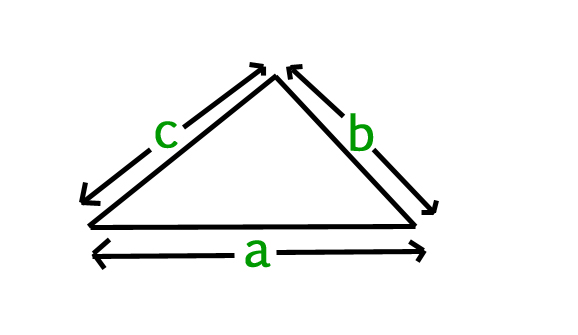# Find Perimeter of a triangle

Given side (a, b, c) of a triangle, we have to find the perimeter of a triangle.

Perimeter :
Perimeter of a triangle is the sum of the length of side of a triangle.where a, b, c are length of side of a triangle.

Perimeter of a triangle can simply be evaluated using following formula :Examples :

```Input : a = 2.0, b = 3.0, c = 5.0
Output : 10.0

Input : a = 5.0, b = 6.0, c = 7.0
Output : 18.0```

## C

 `// A simple C program to find the perimeter ` `// of triangle ` `#include ` ` `  `// Function to find perimeter ` `float` `findPerimeter(``float` `a, ``float` `b, ``float` `c) ` `{ ` `    ``// Formula for finding a perimeter of triangle ` `    ``return` `(a + b + c); ` `} ` ` `  `// Driver Code ` `int` `main() ` `{ ` `    ``float` `a = 2.0, b = 3.0, c = 5.0; ` `    ``printf``(``"%f"``, findPerimeter(a, b, c));   ` `    ``return` `0; ` `} `

## Java

 `// Java program to find perimeter ` `// of triangle ` `class` `Test { ` `    ``static` `float` `findPerimeter(``float` `a, ``float` `b, ``float` `c) ` `    ``{ ` `        ``// Formula for Perimter of triangle ` `        ``return` `(a + b + c); ` `    ``} ` ` `  `    ``// Driver method ` `    ``public` `static` `void` `main(String[] args) ` `    ``{ ` `        ``float` `a = ``2.0``, b = ``3.0``, c = ``5.0``; ` `        ``System.out.println(findPerimeter(a, b, c)); ` `    ``} ` `} `

## Python

 `# Python Program to find a perimeter ` `# of triangle ` ` `  `# Function to find perimeter ` `def` `findPerimeter(a, b, c): ` ` `  `    ``# Calculate the perimeter ` `    ``return` `(a ``+` `b ``+` `c) ` ` `  `# Driver Code     ` `a ``=` `2.0` `b ``=` `3.0` `c ``=` `5.0` `print``(findPerimeter(a, b, c)) `

## C#

 `// C# program to find perimeter ` `// of triangle ` `using` `System; ` ` `  `class` `Test { ` `     `  `    ``static` `float` `findPerimeter(``float` `a,  ` `                           ``float` `b, ``float` `c) ` `    ``{ ` `         `  `        ``// Formula for Perimter of triangle ` `        ``return` `(a + b + c); ` `    ``} ` ` `  `    ``// Driver method ` `    ``public` `static` `void` `Main() ` `    ``{ ` `        ``float` `a = 2.0f, b = 3.0f, c = 5.0f; ` `         `  `        ``Console.WriteLine(findPerimeter(a, b, c)); ` `    ``} ` `} ` ` `  `//This code is contributed by vt_m.  `

## PHP

 ` `

Output :

`10.0`

Attention reader! Don’t stop learning now. Get hold of all the important DSA concepts with the DSA Self Paced Course at a student-friendly price and become industry ready.

My Personal Notes arrow_drop_upCheck out this Author's contributed articles.

If you like GeeksforGeeks and would like to contribute, you can also write an article using contribute.geeksforgeeks.org or mail your article to contribute@geeksforgeeks.org. See your article appearing on the GeeksforGeeks main page and help other Geeks.

Please Improve this article if you find anything incorrect by clicking on the "Improve Article" button below.

Improved By : vt_m